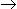# Chemical Engineering - Chemical Reaction Engineering - Discussion

Discussion Forum : Chemical Reaction Engineering - Section 10 (Q.No. 1)
1.
The conversion for a first order liquid phase reaction. AB in a CSTR is 50%. If another CSTR of the same volume is connected in series, then the % conversion at the exit of the second reactor will be
60
75
90
100
Explanation:
No answer description is available. Let's discuss.
Discussion:
4 comments Page 1 of 1.

Aadhar Chauhan said:   3 years ago
Xn=(1-(1/(1+kτ)^n) n= no.of reactor in series having the same volume.
(Space time)τ= V/vá´

Hence, Kτ=0.5/(1-0.5)=1,
(conversion after second reactor)X2= (1-(1/(1+(2^2))) X2=0.75.
(1)

Gaurab Singha Roy said:   6 years ago
Conversion of A in the 1st reactor is 50%. It means when the stream exits 1st reactor CA=0.5. Now if the volume of the in series connected CSTR is same the conversion also will be same i.e. 50%.

So, the exit stream will contain CA = (0.5/2)=0.25. So if initially CAo was 1 and finally it became 0.25, then the total conversion will be 75%.
(2)

Rahul Saini said:   7 years ago
How did you get this answer?

Hikmat said:   9 years ago
I like what you are doing but why don't you have tutorials on the process of mixing in reactors using different impellers, viscosities and different Re numbers.